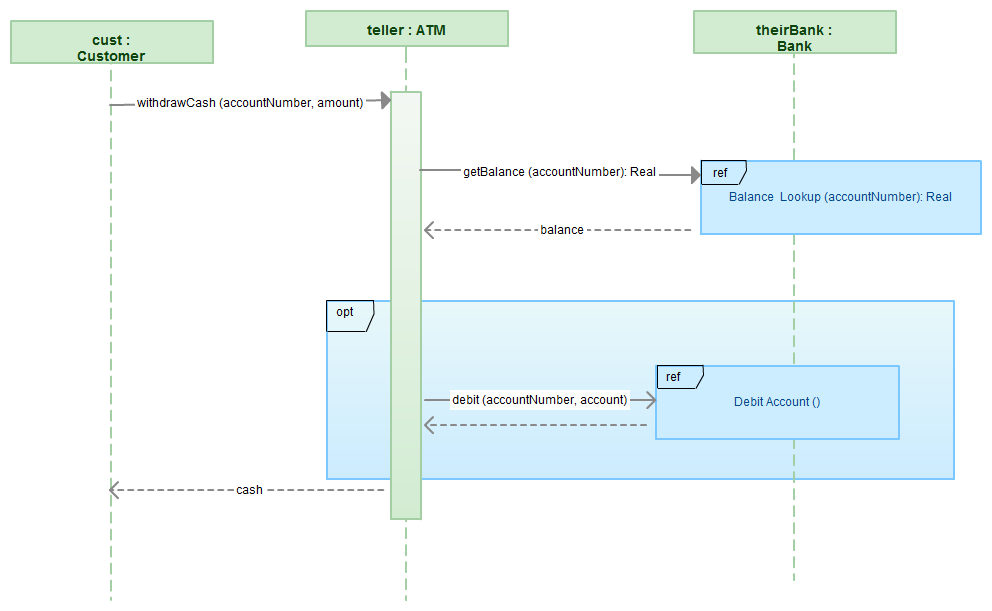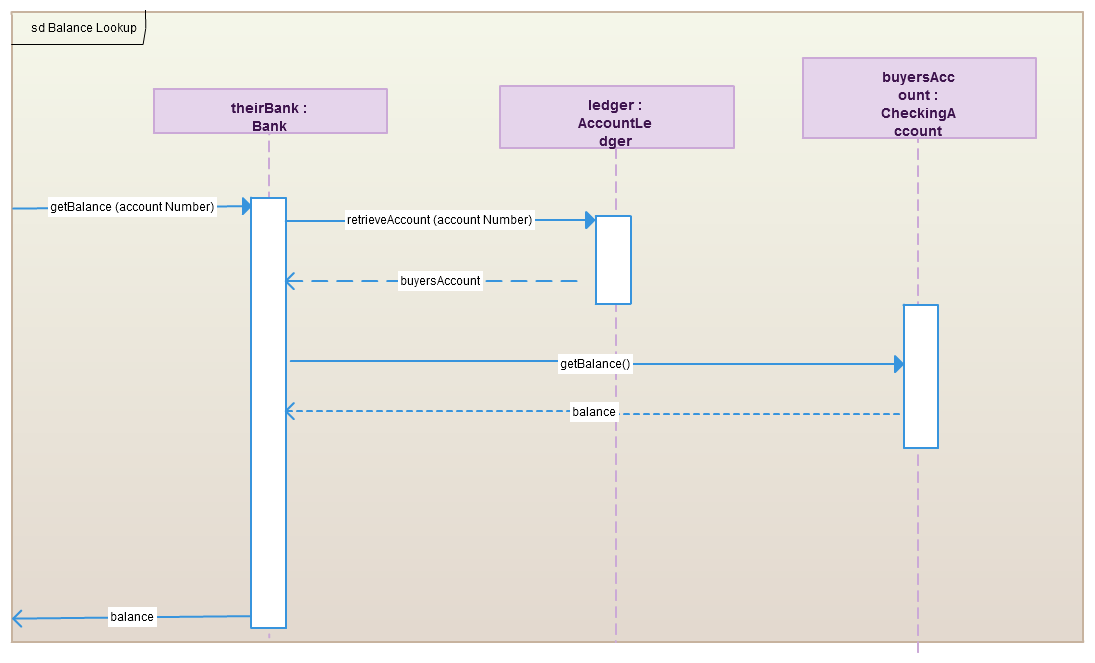# Use of Gates in Sequence Diagrams (Sequence Diagrams Reference)

While we discussed about how to reference another sequence diagram via sending across data through parameters and also return values. Yet, there’s another way to send across data between two sequence diagrams.

Gates could be regarded as being a simple way to model the passing of data between a sequence diagram and its context. A gate is a message that is highlighted with one end connected to the sequence diagram’s frame’s edge and the other end connected to a lifeline.

A reworking of Figure 1 (from How to reference two sequence diagrams) using gates can be seen in the following diagram.

Figure 1Figure 2 (from How to reference two sequence diagrams) with the utilization of gates would look like what is shown below.

Figure 2Figure 2 has an entry gate called getBalance that takes the parameter of accountNumber. The getBalance message is an entry gate, because it is the arrowed line that is linked to the diagram’s frame with the arrowhead linked to a lifeline. The sequence diagram has an exit gate, which returns the balance variable. The exit gate is known, because it’s a return message that is connected from a lifeline to the diagram’s frame with the arrowhead connected to the frame.

Image from - http://www.theoutsourcingcompany.com/blog

Learn How Creately Diagrams works

Learn How Creately Diagrams works